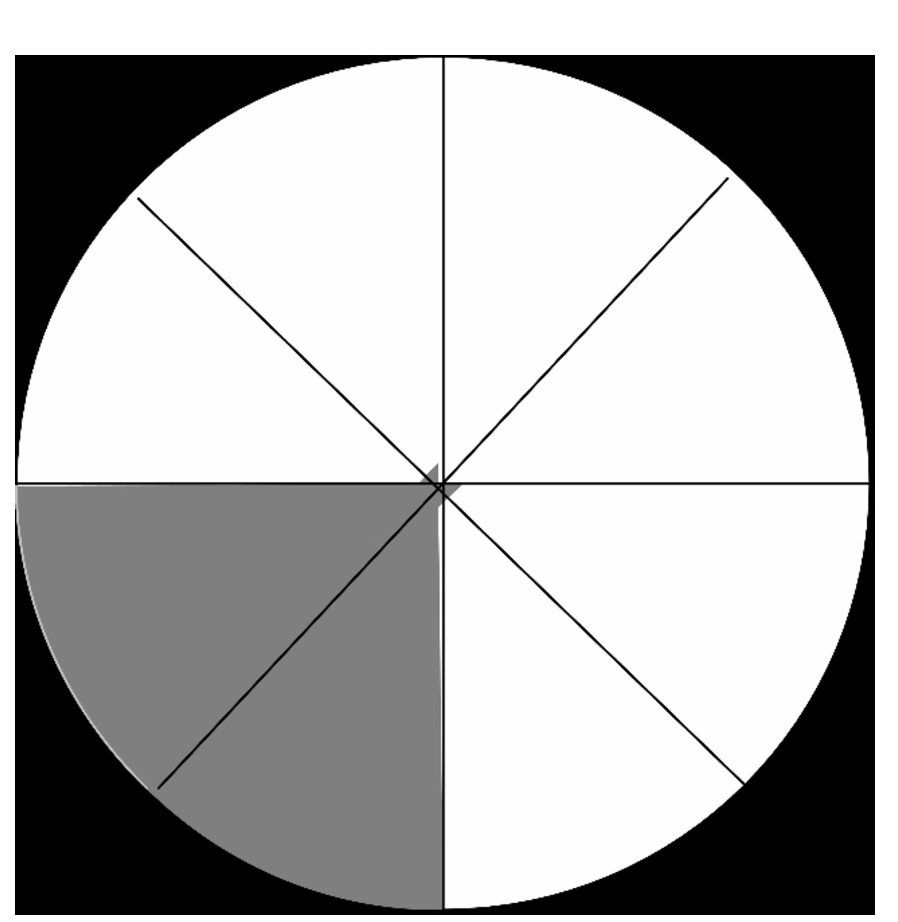top of page

# V.6 #1 - Mathematics - Toward a Deeper Understanding: Strategies to Foster Multiple Meanings of Frac

Brought to you by Learning Disabilities Worldwide (LDW®) through the generosity of Saint Joseph's University.

Knowledge of fractions is such an integral part of the elementary school mathematics curriculum as it provides a foundation for learning measurement, ratio and proportion, percents, probability, and algebra in secondary school mathematics (Clarke, Roche, & Mitchell, 2008; Son, 2011). However, many students have difficulty developing conceptual understanding of fractions. Jones (2012) and Van de Walle, Karp, and Bay-Williams (2010) detail several reasons why fractions are difficult to understand:

• Fraction symbolism is very different from whole number symbolism. In fractional notation, the numerator and denominator are separated by a horizontal line but represent one quantity and not separate values.

• It is difficult to compare the size of fractions. Students can compare whole numbers easily on a number line once they have learned to count meaningfully, but comparing fractions on a number line, for example, requires complex understanding.

• The rules for operations with fractions are different than for whole numbers. For example, students can add 1+2 to get 3 but cannot add 1/2 + 2/3 to get 3/5.

• There are more rules for operations with fractions than for whole numbers and the rules appear contradictory. For example, why can’t you add 1/2 and 2/3 to get 3/5 but you can multiply 1/2 and 2/3 to get 3/6? In the latter, it’s okay to multiply numerators and denominators straight across.

• Students mistakenly believe that parts of the whole do not have to be equal.

Meanings of Fractions

In order to develop a deeper understanding of fractions, it is important to understand all the possible concepts that fractions can represent. In the United States, the most common meaning, and the one taught first, is part-whole. However, there are four meanings that students should understand: part-whole, measure, quotient, and ratio (Jones, 2012). Many who research fraction understanding believe students should understand fractions more deeply with greater emphasis across all meanings of fractions (Siebert & Gaskin, 2006). This article describes the four meanings and strategies teachers can employ to help students gain greater understanding of fractions.

Part-whole. One meaning of fractions is part-whole where a fraction represents a quantity that is partitioned or divided into equal pieces. Imagine that Figure 1 is a pizza which is divided into eight equal slices. Two equal slices eaten (part) out of eight equal slices (the whole) is represented by 2/8.Figure 1. Part-whole meaning of fractions.

Measure. Fractions can also be represented as a comparison of lengths. A length is identified and then is used as the unit for comparison. In Figure 2, the fraction 2/8 can be measured relative to the length of the whole or can be considered as two copies of the length 1/8.Figure 2. Fraction represented as a measure. Quotient. A fraction can represent the division of two numbers. If I had two cookies and eight people equally sharing the cookies, each person would receive 2 ÷ 8 or 2/8 of a cookie. Ratio. A fraction can compare two sets or measurements. Ratios can be part-part or part-whole. Let’s say there are two boys for every eight girls in a third grade class, the ratio of boys (part) to girls (part) would be 2:8 which can be represented as 2/8. Let’s say that in a fourth grade class two boys have striped shirts (part) out of a class of eight students (whole), the ratio can be written as 2/8. Models for Understanding Fractions

There are several models to assist students in understanding the meanings of fractions. Different models provide different opportunities to learn; when instructing students about fractions, encourage them to use models for as long as necessary. Eventually, they may be able to use mental models of fractions instead of concrete or pictorial models. Three types of models discussed in this article are: area/region model, length/measurement model, and set model.

Area/region model. Area or region models help students to visualize part of a whole. Some commonly used manipulatives to assist students with this model are:

• Circular fraction pieces (Figure 3). These “fraction circles” allow students to see the size of parts relative to the whole. Students can stack the pieces on top of one another to compare sizes of fractions. They can also be used to teach equivalent fractions and basic addition and subtraction of fractions.Figure 3. Circular fraction pieces.

• Paper folding. Paper is a great tool for students to begin to explore part-whole relationships. One benefit of using paper is that it is generally readily available in schools unlike some of the more “fancy” manipulatives. Have students fold pieces of paper to create different fractions (e.g., halves